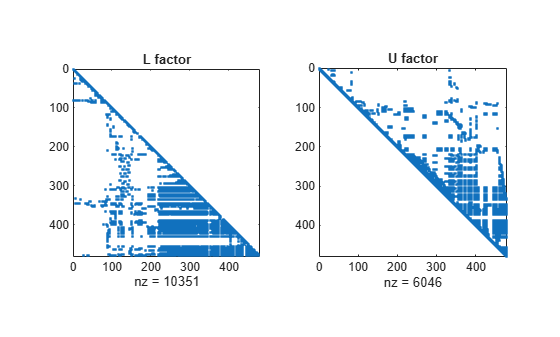# lu

LU 矩阵分解

## 语法

``[L,U] = lu(A)``
``[L,U,P] = lu(A)``
``[L,U,P] = lu(A,outputForm)``
``[L,U,P,Q] = lu(S)``
``[L,U,P,Q,D] = lu(S)``
``[___] = lu(S,thresh)``
``[___] = lu(___,outputForm)``

## 说明

``[L,U] = lu(A)` 将满矩阵或稀疏矩阵 `A` 分解为一个上三角矩阵 `U` 和一个经过置换的下三角矩阵 `L`，使得 `A = L*U`。`

``[L,U,P] = lu(A)` 还返回一个置换矩阵 `P`，并满足 `A = P'*L*U`。在此语法中，`L` 是单位下三角矩阵，`U` 是上三角矩阵。`

``[L,U,P] = lu(A,outputForm)` 以 `outputForm` 指定的格式返回 `P`。将 `outputForm` 指定为 `'vector'` 会将 `P` 返回为一个置换向量，并满足 `A(P,:) = L*U`。`

``[L,U,P,Q] = lu(S)` 将稀疏矩阵 `S` 分解为一个单位下三角矩阵 `L`、一个上三角矩阵 `U`、一个行置换矩阵 `P` 以及一个列置换矩阵 `Q`，并满足 `P*S*Q = L*U`。`
``[L,U,P,Q,D] = lu(S)` 还返回一个对角缩放矩阵 `D`，并满足 `P*(D\S)*Q = L*U`。行缩放通常会使分解更为稀疏和稳定。`
``[___] = lu(S,thresh)` 可结合上述任意输出参数组合指定 `lu` 使用的主元消去策略的阈值。根据指定的输出参数的数量，对 `thresh` 输入的要求及其默认值会有所不同。有关详细信息，请参阅 `thresh` 参数说明。`

``[___] = lu(___,outputForm)` 以 `outputForm` 指定的格式返回 `P` 和 `Q`。将 `outputForm` 指定为 `'vector'` 以将 `P` 和 `Q` 返回为置换向量。您可以使用上述语法中的任何输入参数组合。`

## 示例

```A = [10 -7 0 -3 2 6 5 -1 5];```
`[L,U] = lu(A)`
```L = 3×3 1.0000 0 0 -0.3000 -0.0400 1.0000 0.5000 1.0000 0 ```
```U = 3×3 10.0000 -7.0000 0 0 2.5000 5.0000 0 0 6.2000 ```

`L*U`
```ans = 3×3 10.0000 -7.0000 0 -3.0000 2.0000 6.0000 5.0000 -1.0000 5.0000 ```

`[L,U,P] = lu(A)`
```L = 3×3 1.0000 0 0 0.5000 1.0000 0 -0.3000 -0.0400 1.0000 ```
```U = 3×3 10.0000 -7.0000 0 0 2.5000 5.0000 0 0 6.2000 ```
```P = 3×3 1 0 0 0 0 1 0 1 0 ```
`P'*L*U`
```ans = 3×3 10.0000 -7.0000 0 -3.0000 2.0000 6.0000 5.0000 -1.0000 5.0000 ```

```A = magic(5); b = 65*ones(5,1); x = A\b```
```x = 5×1 1.0000 1.0000 1.0000 1.0000 1.0000 ```

```y = L\(P*b); x = U\y; ```

`[L,U,P] = lu(A)`
```L = 5×5 1.0000 0 0 0 0 0.7391 1.0000 0 0 0 0.4783 0.7687 1.0000 0 0 0.1739 0.2527 0.5164 1.0000 0 0.4348 0.4839 0.7231 0.9231 1.0000 ```
```U = 5×5 23.0000 5.0000 7.0000 14.0000 16.0000 0 20.3043 -4.1739 -2.3478 3.1739 0 0 24.8608 -2.8908 -1.0921 0 0 0 19.6512 18.9793 0 0 0 0 -22.2222 ```
```P = 5×5 0 1 0 0 0 1 0 0 0 0 0 0 0 0 1 0 0 1 0 0 0 0 0 1 0 ```
```y = L\(P*b); x = U\y```
```x = 5×1 1.0000 1.0000 1.0000 1.0000 1.0000 ```

```dA = decomposition(A,'lu'); x = dA\b```
```x = 5×1 1.0000 1.0000 1.0000 1.0000 1.0000 ```

`S = bucky;`

`[L,U,P,Q] = lu(S);`

```e = P*S*Q - L*U; norm(e,1)```
```ans = 5.7732e-15 ```

`A = rand(1000);`

```[L1,U1,P] = lu(A); [L2,U2,p] = lu(A,'vector'); whos P p```
``` Name Size Bytes Class Attributes P 1000x1000 8000000 double p 1x1000 8000 double ```

```load west0479 A = west0479;```

```[L,U,P] = lu(A); subplot(1,2,1) spy(L) title('L factor') subplot(1,2,2) spy(U) title('U factor')``````[L,U,P,Q] = lu(A); subplot(1,2,1) spy(L) title('L factor') subplot(1,2,2) spy(U) title('U factor')```## 输入参数

• 当输出为三个或少于三个时，`thresh` 必须是标量，默认值为 `1.0`

• 当输出为四个或多于四个时，`thresh` 可以是标量或二元素向量。默认值为 `[0.1 0.001]`。如果将 `thresh` 指定为标量，则仅替换向量中的第一个值。

`lu` 首先根据输出参数的数量、然后根据待分解矩阵的属性选择主元消去策略。在任何情况下，将阈值设置为 `1.0` 都会导致部分主元消去，而将它们设置为 `0` 则会导致仅根据生成的矩阵的稀疏性选择主元。`L` 的所有值的绝对值都小于或等于 `1/min(thresh)`

• 三个或少于三个的输出参数 - 如果算法满足以下等式，算法会选择对角主元

`A(j,j) >= thresh * max(abs(A(j:m,j)))`
否则，它会选择包含绝对值最大的元素所在的行。

• 对称主元消去策略 - 如果 `S` 是大体结构对称和对角线上元素多为非零元素的稀疏方阵，则 `lu` 将使用对称主元消去策略。对于此策略，如果算法满足以下不等式，则算法会选择对角主元 `j`

`A(i,j) >= thresh(2) * max(abs(A(j:m,j)))`
如果对角线元未通过此测试，则 `lu` 会选择满足以下不等式的最稀疏的行 `i`
`A(i,j) >= thresh(1) * max(abs(A(j:m,j)))`

• 非对称主元消去策略 - 如果 `S` 不满足对称主元消去策略的要求，则 `lu` 将使用非对称策略。在这种情况下，`lu` 会选择满足以下不等式的最稀疏的行 `i`

`A(i,j) >= thresh(1) * max(abs(A(j:m,j)))`
对于 `thresh(1)`，值为 `1.0` 会执行传统的部分主元消去。`L` 中的元的绝对值都小于或等于 `1/thresh(1)`。当使用非对称策略时，不会使用 `thresh` 输入向量的第二个元素。

• 三个输出 - `P` 满足 `P*A = L*U`

• 四个输出 - `P``Q` 满足 `P*S*Q = L*U`

• 五个输出 - `P``Q``D` 满足 `P*(D\S)*Q = L*U`

• 三个输出 - `P` 满足 `A(P,:) = L*U`

• 四个输出 - `P``Q` 满足 `S(P,Q) = L*U`

• 五个输出 - `P``Q``D` 满足 `D(:,P)\S(:,Q) = L*U`

## 输出参数

• 如果指定第三个输出 `P`，则 `L` 返回为一个单位下三角矩阵（即，主对角线上元素为 1 的下三角矩阵）。

• 如果未指定第三个输出 `P`，则 `L` 返回为一个单位下三角矩阵的行置换矩阵。具体而言，它是三输出情况下所返回输出 `P``L``P'*L` 的乘积。

## 算法

LU 分解是使用 `inv` 得到逆矩阵和使用 `det` 得到行列式的关键步骤。同时，它还是使用运算符 `\``/` 求线性方程解或矩阵除法的基础。这意味着 `lu` 的这些数值限制也会存在于依赖它的这些函数中。